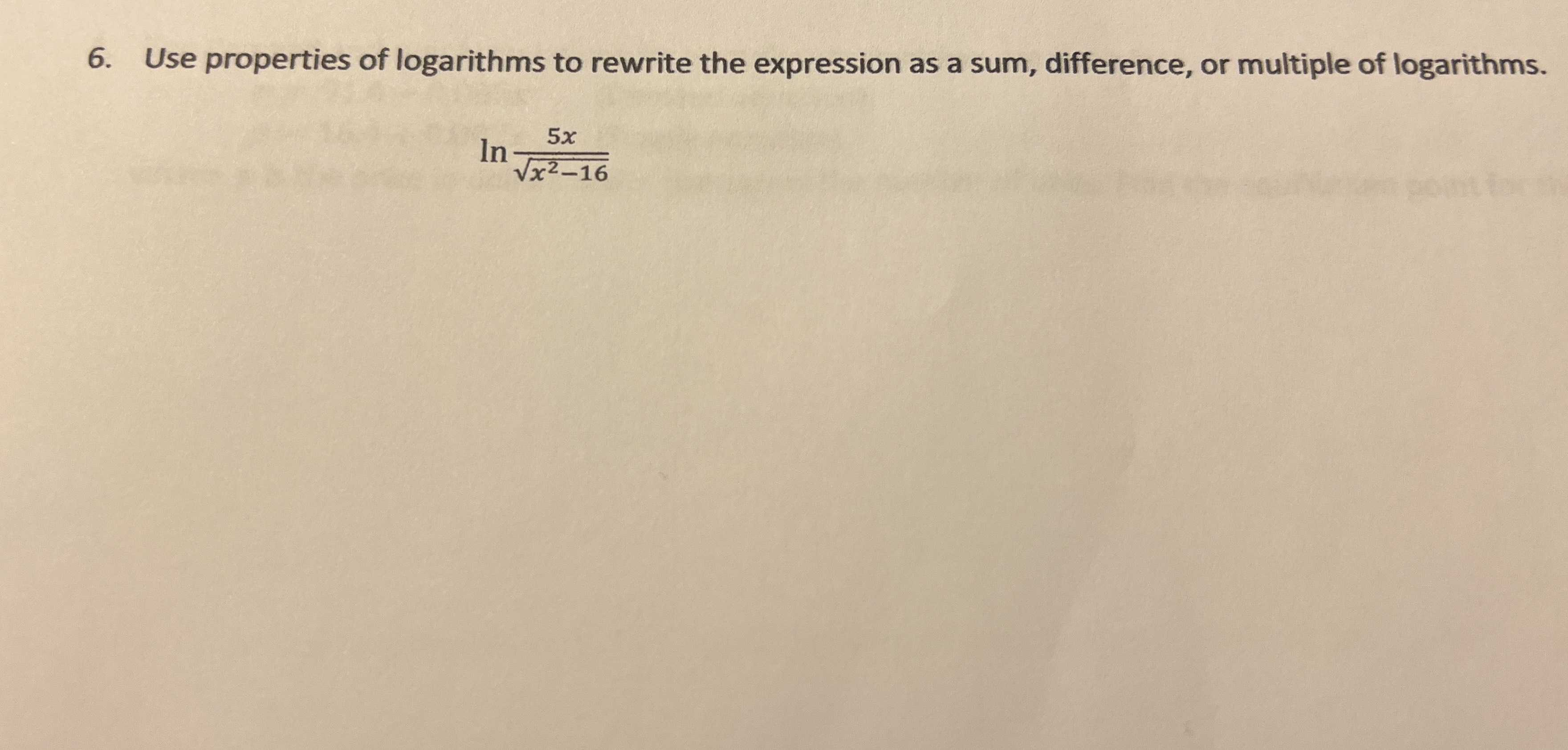### ¿Todavía tienes preguntas de matemáticas?

Pregunte a nuestros tutores expertos
Algebra
Pregunta6. Use properties of logarithms to rewrite the expression as a sum, difference, or multiple of logarithms.

$$\ln \frac { 5 x } { \sqrt { x ^ { 2 } - 16 } }$$

$$ln5+ lnx- \frac{1}{2}ln(x^{2} - 16)$$